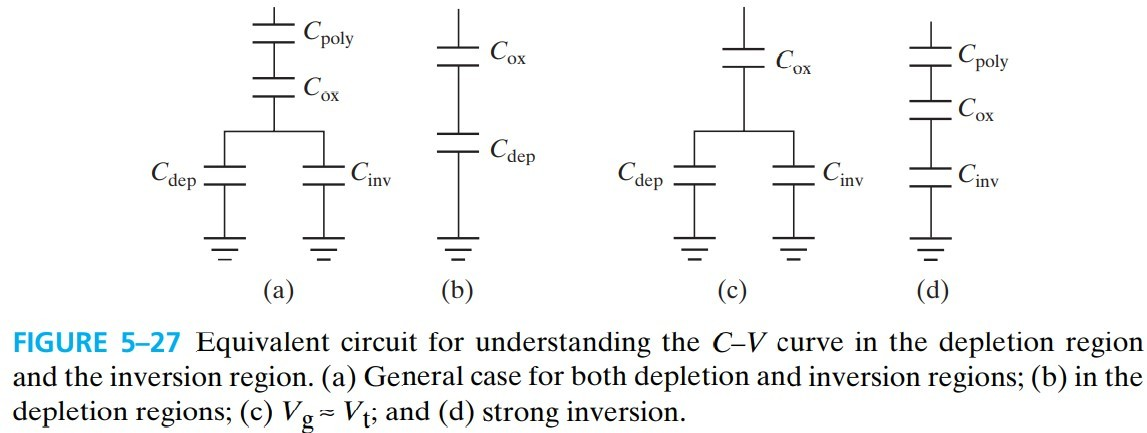# MOS Capacitor

\begin{align*} \newcommand{\dif}{\mathop{}\!\mathrm{d}} \newcommand{\belowarrow}{\mathop{#1}\limits_{\uparrow}} \newcommand{\bd}{\boldsymbol} \newcommand{\L}{\mathscr{L}} \end{align*}

MOS 代表的是 metal-oxide-semionductor，一个典型的 MOS 由三部分组成：

• semiconductor substrate
• insulator film
• metal electrode - called gate

MOS Capacitor MOS Transistor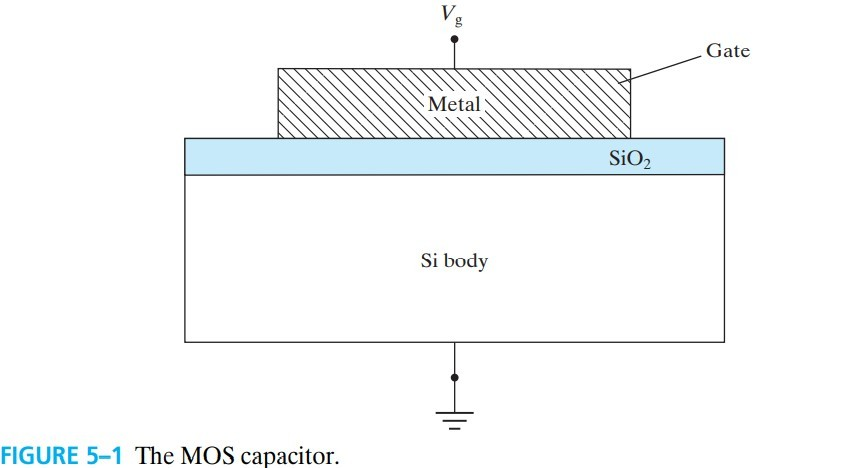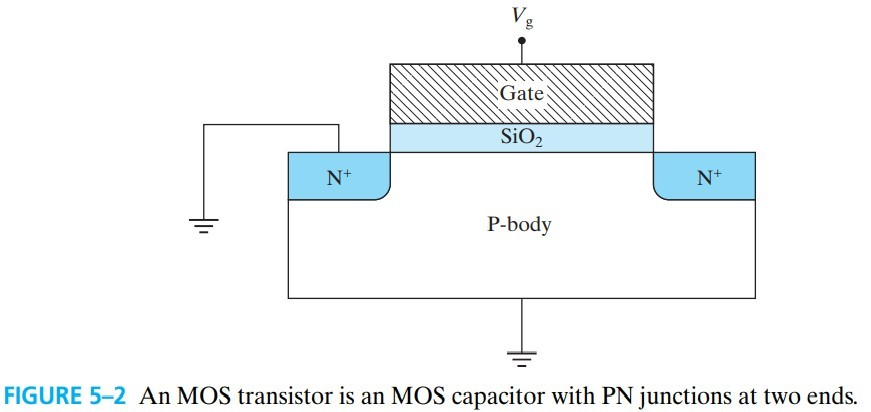</center>

# 5.1 Flat-Band Condtion and Flat-Band Voltage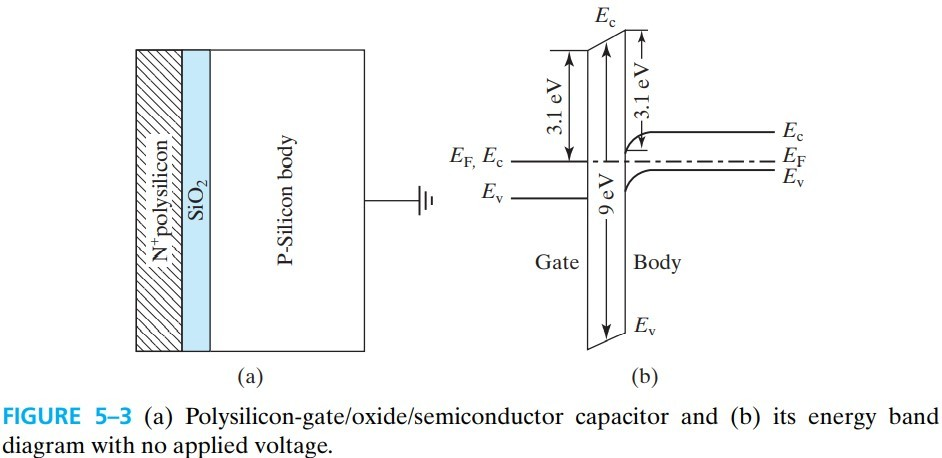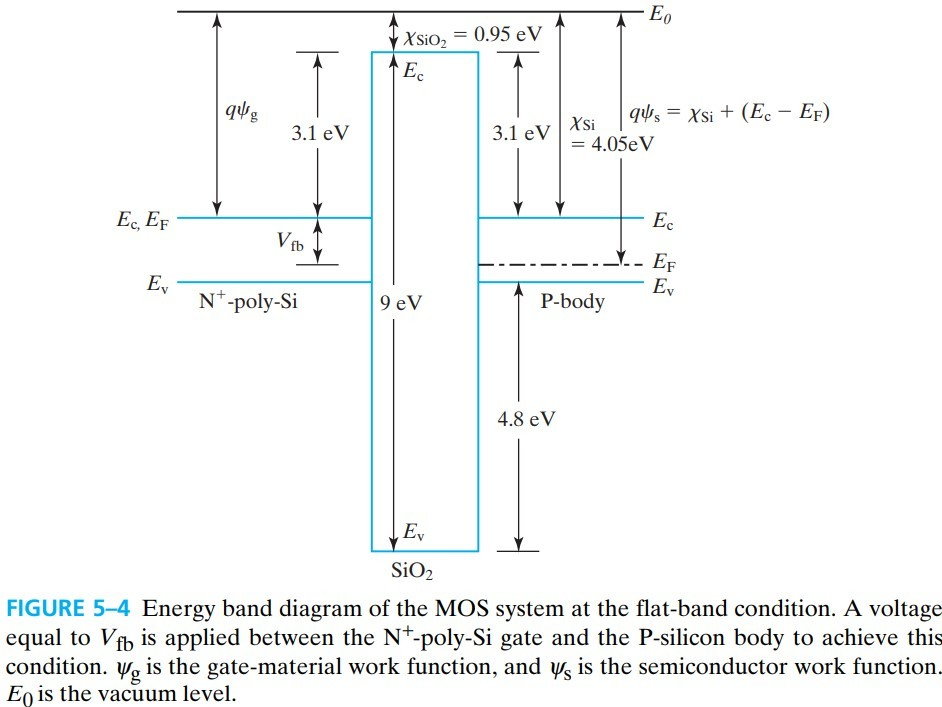1. flat-band condition 是通过在 gate 上加负电压得到的。因为只有加负压才能提高电势。
2. 因为能带是平的，所以
• substrate 的 the surface electric field 为 0
• ${\rm SiO_2}$ 中的 $E_c,E_v$ 也为 0，且 $E_g=9 \text{eV}$
3. $E_0$ 是 vacuum level，the energy state of electrons outside the material
4. 图中几个差值：
• $E_0-E_c=\chi$ 称为 电子亲合能 electron affinity，是一个材料的固定参数
• ${\rm Si}$ 的 $E_c$ 与 ${\rm SiO_2}$ 的 $E_c$ 之差称为 electron energy barrier
• ${\rm Si}$ 的 $E_v$ 与 ${\rm SiO_2}$ 的 $E_v$ 之差称为 hole energy barrier
5. 为了简化分析，${\rm N^+ \; poly \; Si}$ 的 $E_F$ 与 $E_c$ 重合
6. 因为 ${\rm SiO_2}$ 的禁带很宽，所以 $E_F$ 放中间哪个位置都差不多，我们干脆就不管它了

$V_\text{fb} = \psi_g - \psi_s \tag{5.1.1}\\ 考试请使用 (5.7.1) 的修正公式$

$\psi_g,\psi_s$ 是 gate and semi’ 的真空能级与费米能级之间的差值，称为 work function

# 5.2 Surface Accumulation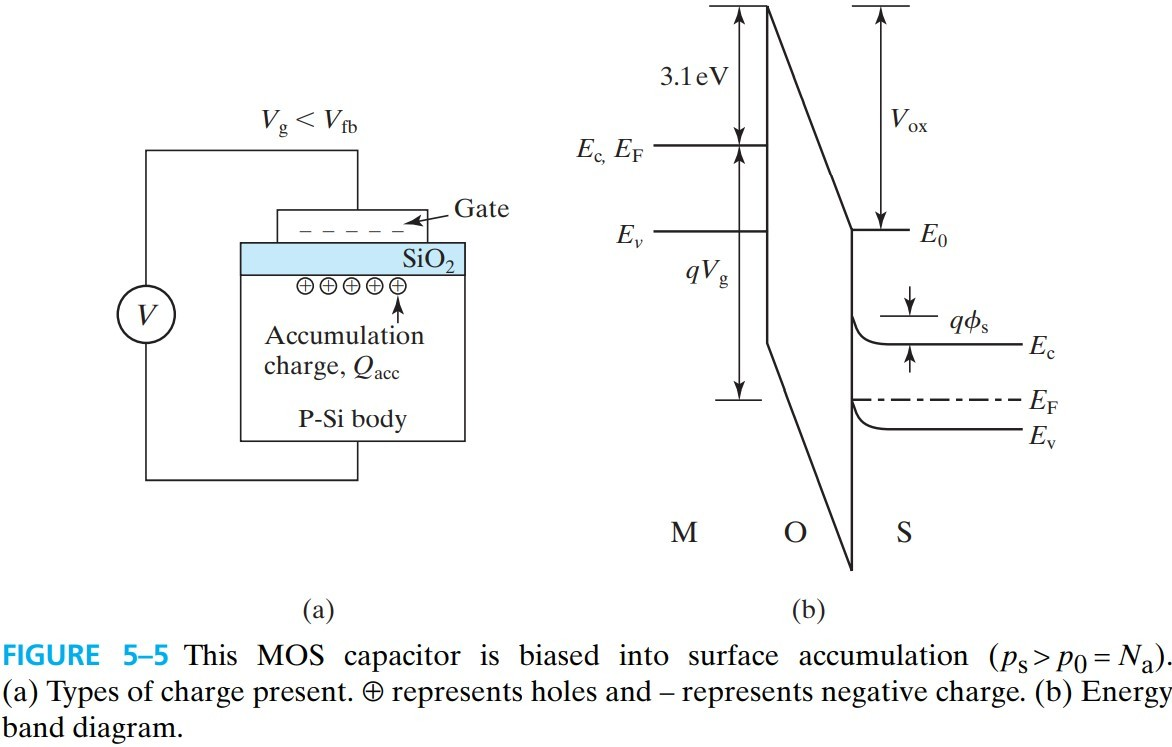1. gate 的能带没弯，说明它上面几乎没电压降（毕竟金属内部没电场）
2. ${\rm SiO_2}$ 上的电压 oxide voltage 为 $V_\text{ox}$，定义为 gate 减去 substrate，所以是 up toward the gate is negative（图中）
3. substrate 的电压降为 $\phi_s$，并且主要集中在表面。$\phi_s$ 是 surface voltage，因为衬底是 0 电势，所以 bends upward is negative（图中），bends downword is positive.

$p_s = N_a e^{-q \phi_s / kT} \tag{5.2.1}$

$V_g = V_\text{fb}+\phi_s+V_\text{ox} \tag{5.2.2}$

$\mathscr{E}_\text{ox} = -\frac{Q_\text{acc}}{\varepsilon_\text{ox}}$

$V_\text{ox} = \mathscr{E}_\text{ox} T_\text{ox} = -\frac{Q_\text{acc}}{C_\text{ox}} \tag{5.2.4}$

$Q_\text{acc}=-C_\text{ox}(V_g-V_\text{fb}-\phi_s) \tag{5.2.5a}$

$Q_\text{acc}=-C_\text{ox}(V_g-V_\text{fb}) \tag{5.2.5b}$

$V_\text{ox}=-Q_\text{sub}/C_\text{ox}$

# 5.3 Surface Depletion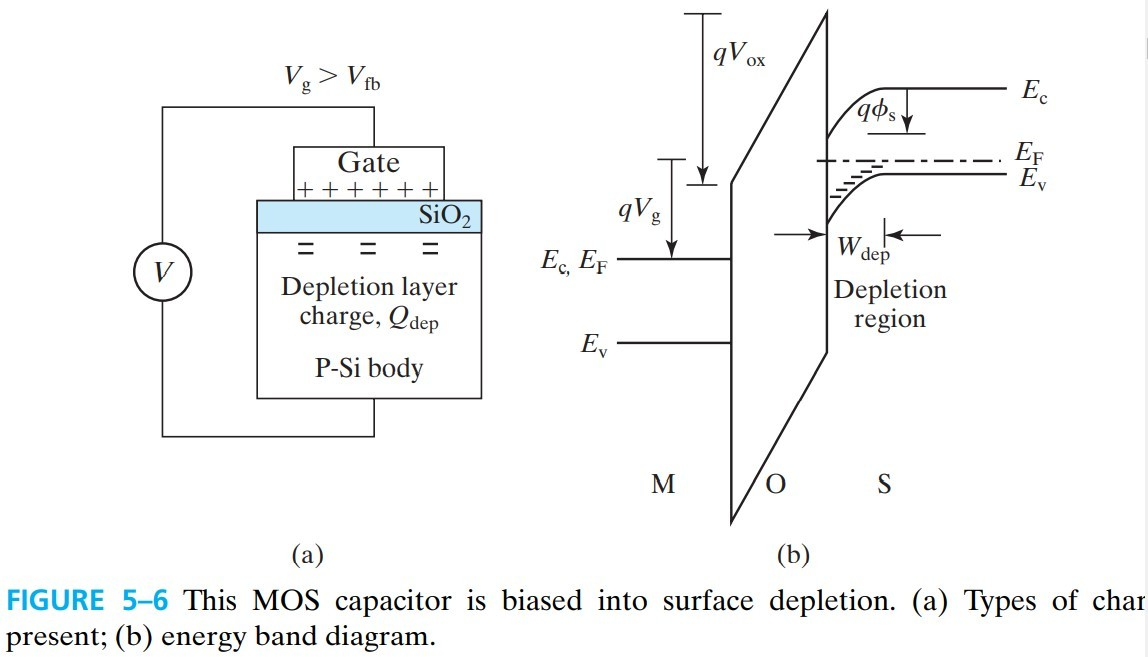$V_\text{ox}=-\frac{Q_\text{sub}}{C_\text{ox}}=-\frac{Q_\text{dep}}{C_\text{ox}}=\frac{q N_a W_\text{dep}}{C_\text{ox}}$

$V_\text{ox}= \frac{\sqrt{q N_a 2 \varepsilon_s \phi_s}}{C_\text{ox}} \tag{5.3.1}$ $以及 \; \phi_s = \frac{q N_a W_\text{dep}^2}{2 \varepsilon_s} \tag{5.3.2}$

$V_g = V_\text{fb}+\phi_s+V_\text{ox}=V_\text{fb}+\frac{q N_a W_\text{dep}^2}{2 \varepsilon_s}+\frac{q N_a W_\text{dep}}{C_\text{ox}} \tag{5.3.3}$

# 5.4 Threshold Condition and Threshold Voltage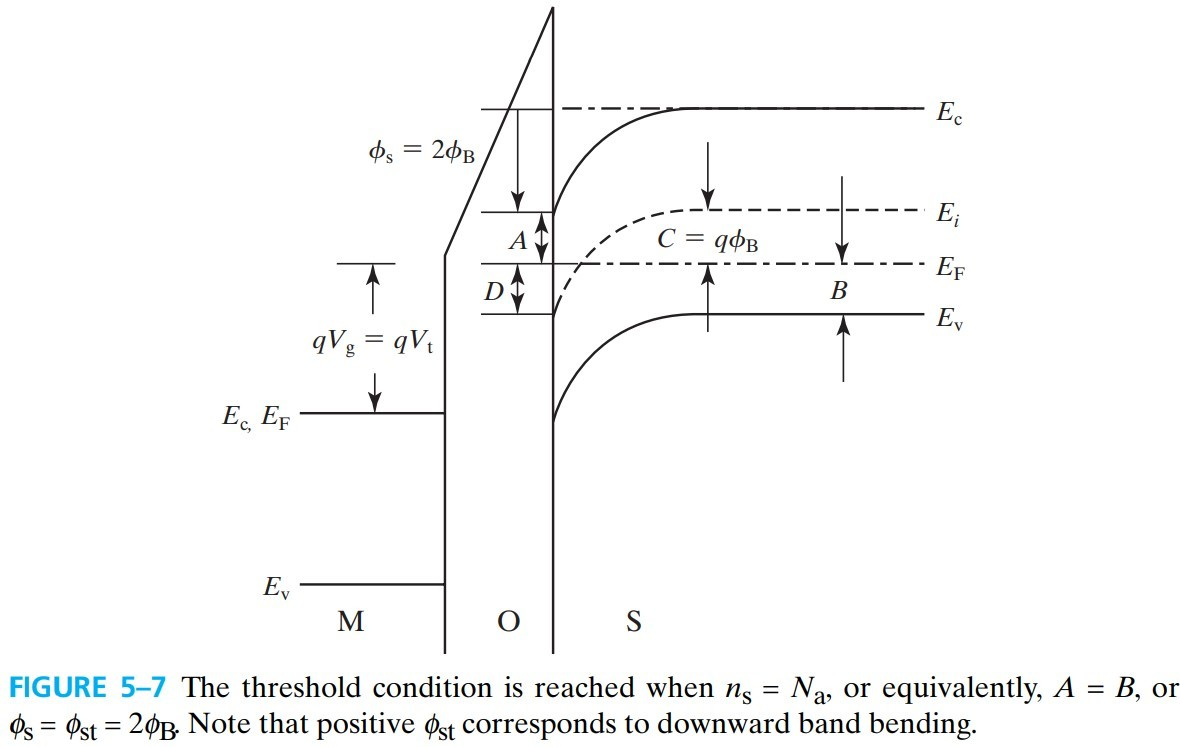\begin{aligned} q\phi_B &\equiv \left. \frac{E_g}{2}-(E_F-E_v) \right\vert_\text{bulk}\\ &=k T \ln \frac{N_v}{n_i}-kT \ln \frac{N_v}{N_a}\\ &=kT\ln \frac{N_a}{n_i} \end{aligned} \tag{5.4.1}

$\phi_{st} = 2 \phi_B = 2 \frac{kT}{q} \ln \frac{N_a}{n_i} \tag{5.4.2}$

\begin{aligned} V_t &= V_\text{fb}+\phi_s+V_\text{ox}\\ &= V_\text{fb}+2 \phi_B+\frac{\sqrt{q N_a 2 \varepsilon_s 2\phi_B}}{C_\text{ox}} \end{aligned} \tag{5.4.3}

$$V_t = V_\text{fb}+\phi_{st}-\frac{\sqrt{2 q N_d \varepsilon_s |\phi_{st}|}}{C_\text{ox}}$$ $$\phi_{st}=-2\phi_B$$ $$\phi_B=\frac{kT}{q}\ln \frac{N_d}{n_i}$$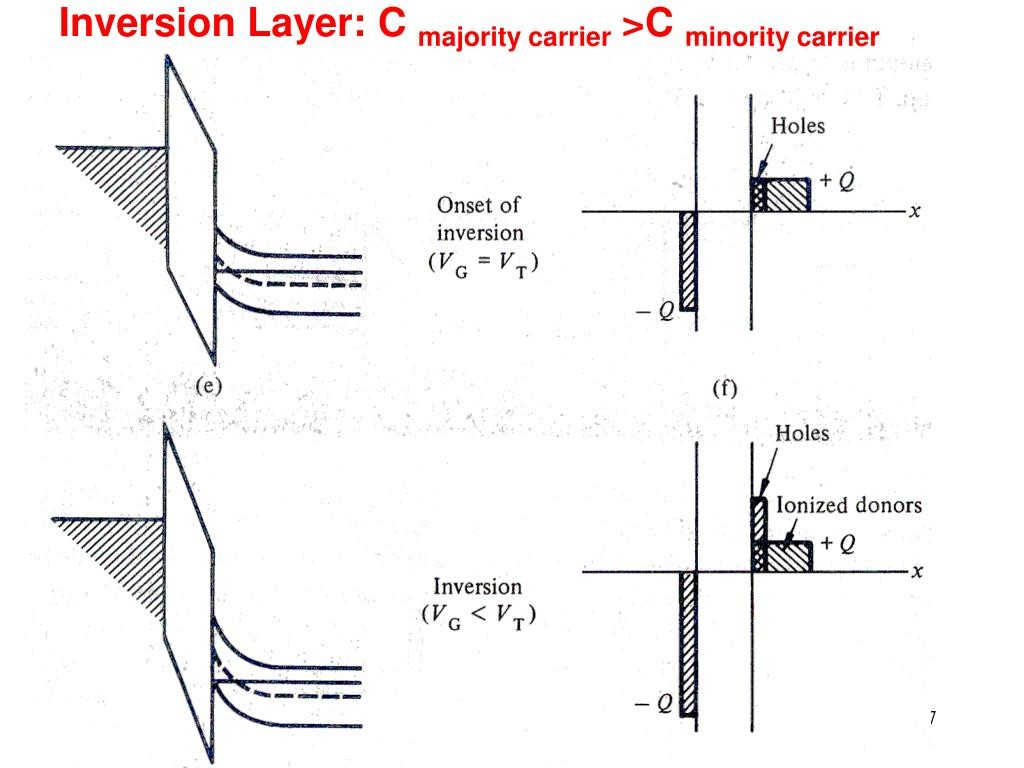# 5.5 Strong Inversion Beyond Threshold$W_\text{dep} = \sqrt{\dfrac{2 \varepsilon_s \phi_s}{qN_a}}\\ \Rightarrow W_\text{dmax} = \sqrt{\dfrac{2 \varepsilon_s 2 \phi_B}{qN_a}} \tag{5.5.1}$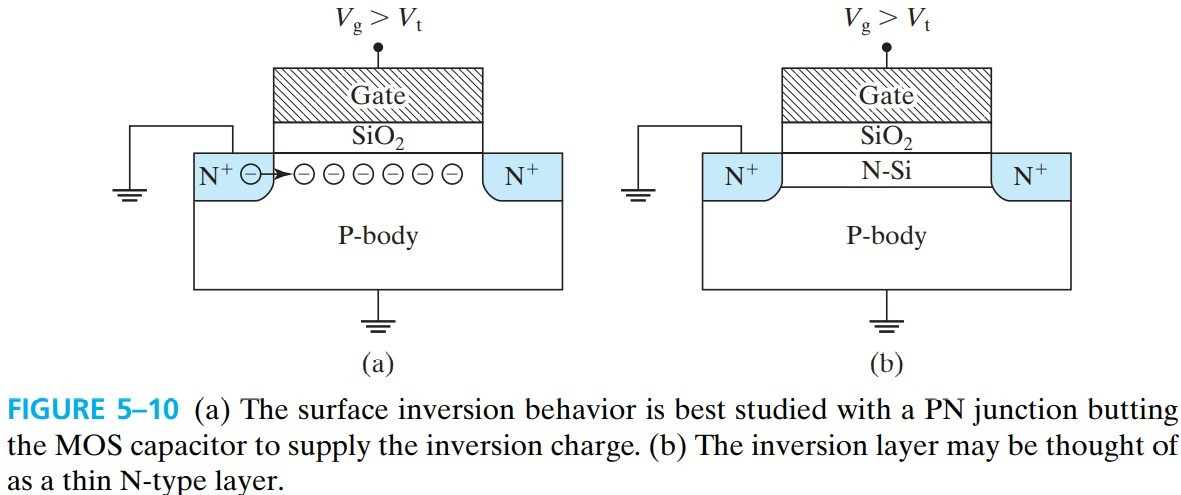\begin{aligned} V_g &= V_\text{fb}+2\phi_B-\frac{Q_\text{dep}}{C_\text{ox}}\\ &=V_\text{fb}+2\phi_B+\frac{\sqrt{q N_a 2 \varepsilon+s 2 \phi_B}}{C_\text{ox}}-\frac{Q_\text{inv}}{C_\text{ox}}\\ &=V_t-\frac{Q_\text{inv}}{C_\text{ox}} \end{aligned} \tag{5.5.2}

$Q_\text{inv} = - C_\text{ox}(V_g-V_t) \tag{5.5.3}$

## 5.5.1 Choice of Vt and Gate Doping Type

MOS 一般都是工作在 $V_t$ 附近，所以我们希望这个电压最好小点，因此，p衬底要用 ${\rm P^+}$ gate，n衬底要用 ${\rm N^+}$ gate.（书上并没有进一步解释）

# 小结

• surface accumulation $V_g<V_\text{fb}$
• flat-Band condtion $V_g=V_\text{fb}$
• surface depletion $V_\text{fb}<V_g<V_t$
• threshold of inversion $V_g=V_t$
• strong inversion $V_g>V_t$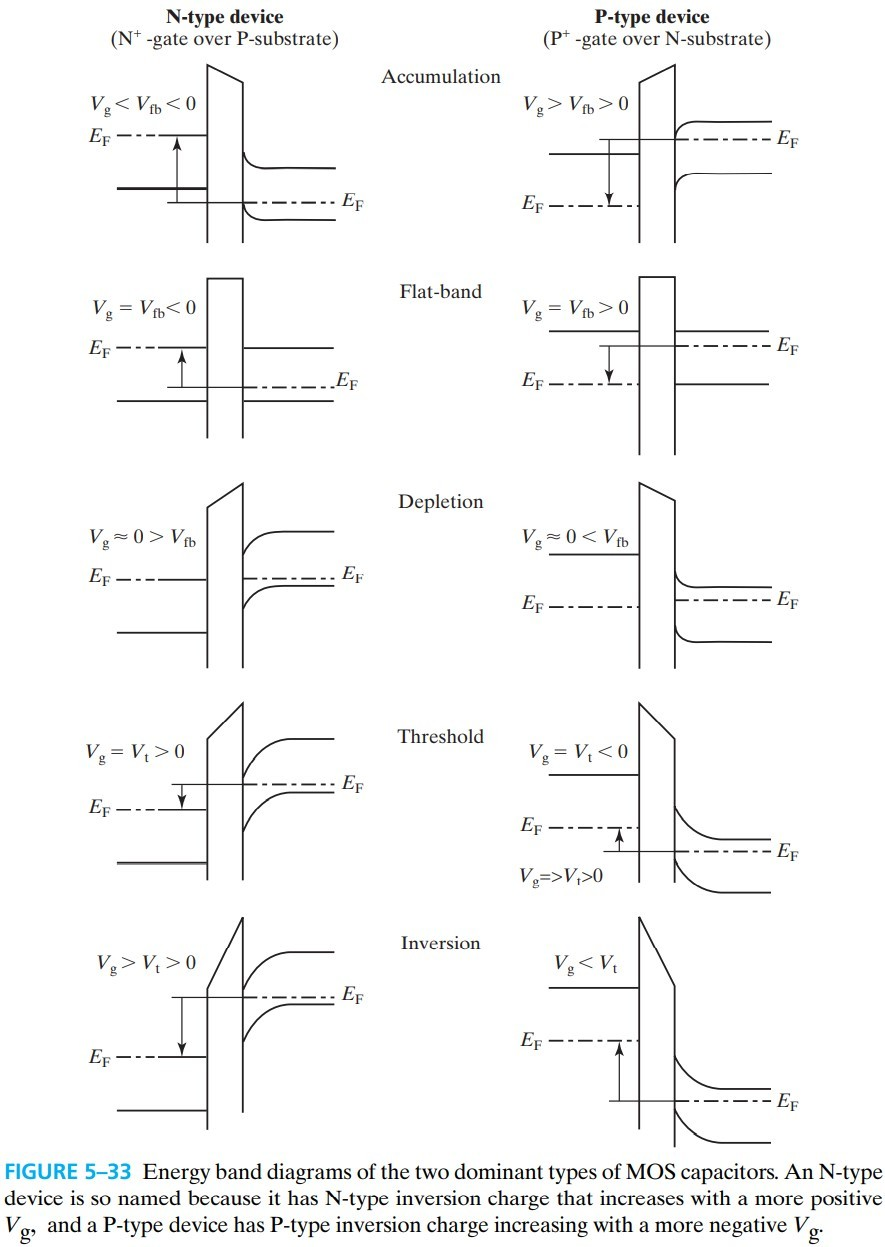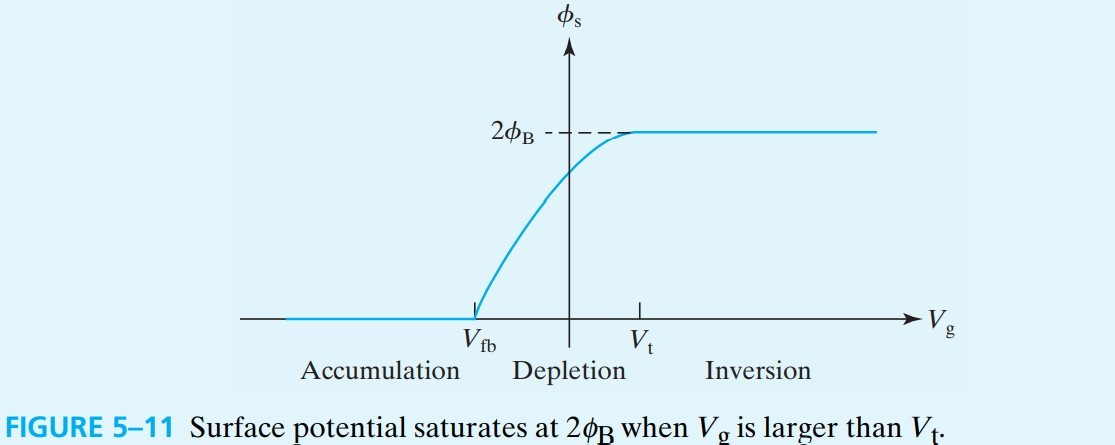$\phi_s$ 的变化情况见上图，$W_\text{dep}$，$Q_\text{acc},Q_\text{dep},Q_\text{inv}$ 的变化情况见下图：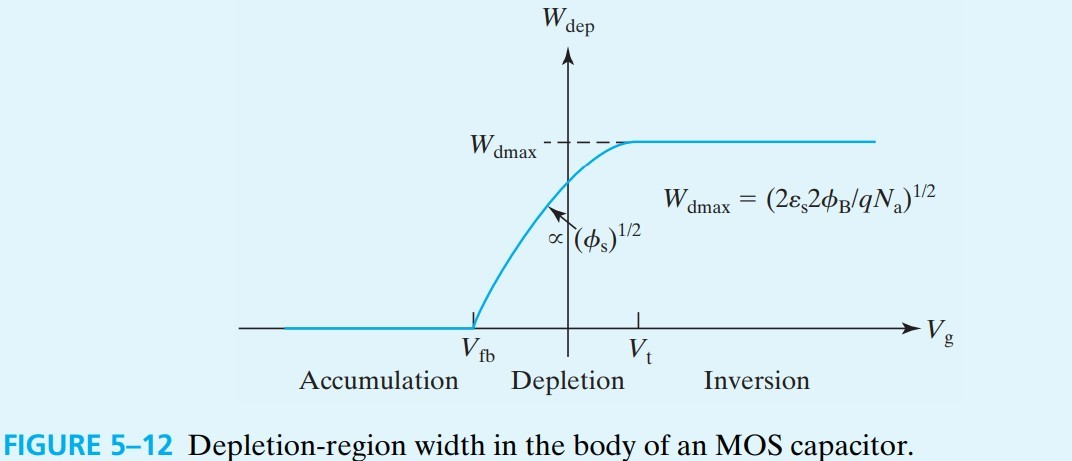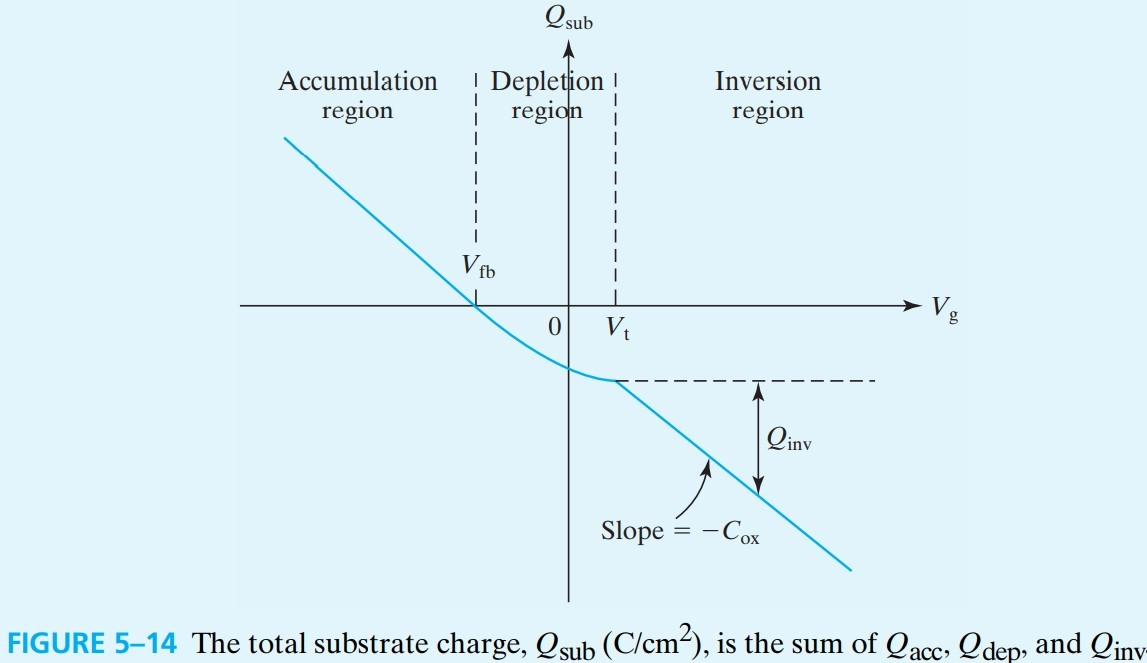# 5.6 MOS C-V Characteristics

$C \equiv \frac{\dif Q_g}{\dif V_g} = -\frac{\dif Q_\text{sub}}{\dif V_g} \tag{5.6.1}$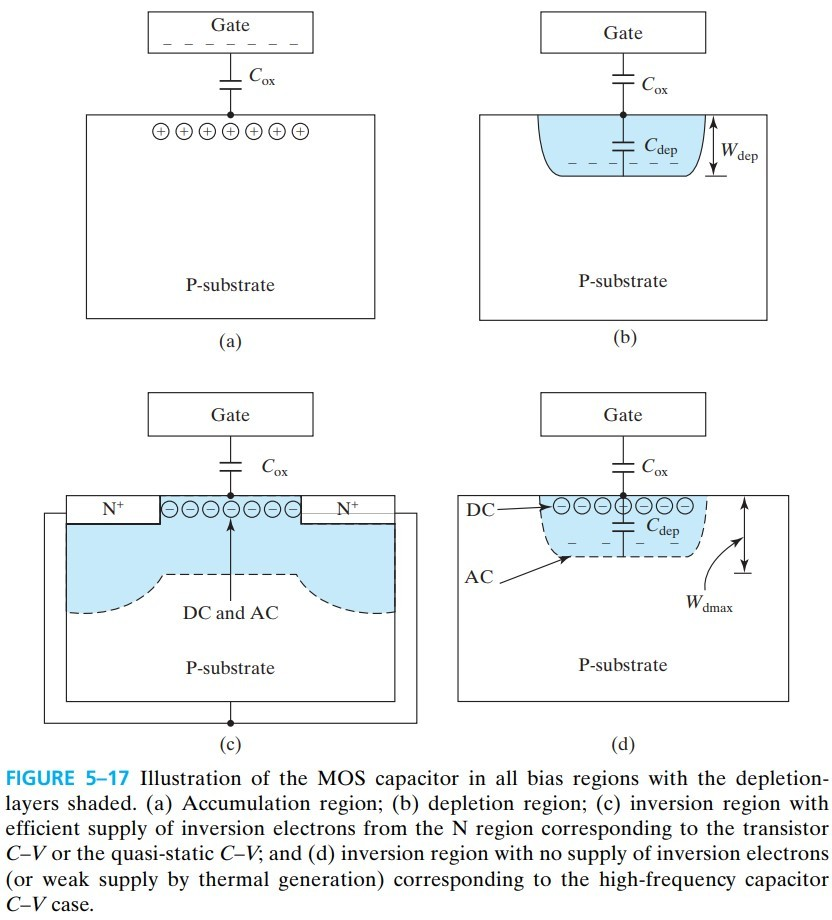$C_\text{dep}=\frac{\varepsilon_s}{W_\text{dep}} \tag{5.6.2}$ $\frac{1}{C}=\frac{1}{C_\text{ox}}+\frac{1}{C_\text{dep}} \tag{5.6.3}$

\begin{aligned} V_g &= V_\text{fb}+ \frac{q N_a W_\text{dep}^2}{2 \varepsilon_s}+\frac{q N_a W_\text{dep}}{C_\text{ox}}\\ &\Rightarrow \frac{2(V_g-V_\text{fb})}{q N_a \varepsilon_s}=\frac{1}{C_\text{dep}^2}+\frac{2}{C_\text{ox} C_\text{dep}} \\ &\Rightarrow \frac{2(V_g-V_\text{fb})}{q N_a \varepsilon_s}+\frac{1}{C_\text{ox}^2}=(\frac{1}{C_\text{dep}}+\frac{1}{C_\text{ox}})^2\\ &\Rightarrow \frac{1}{C}=\sqrt{\frac{2(V_g-V_\text{fb})}{q N_a \varepsilon_s}+\frac{1}{C_\text{ox}^2}} \end{aligned}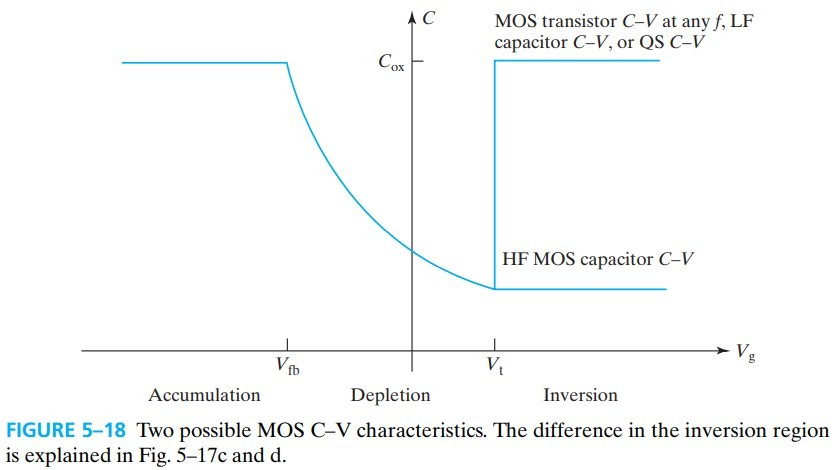# 5.7 Oxide Charge - A Modification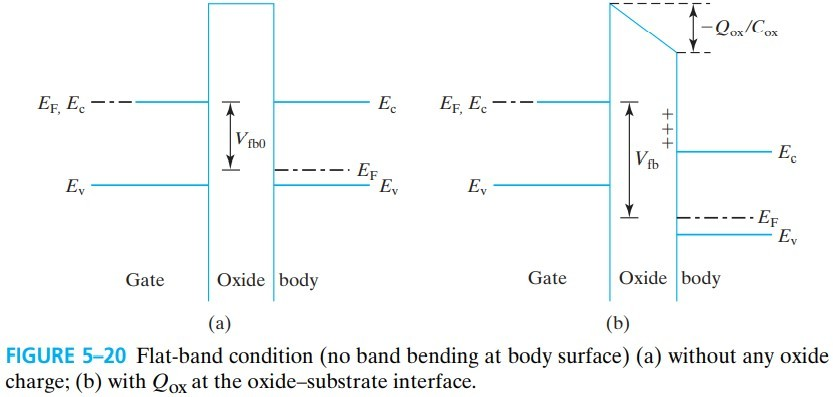\begin{aligned} V_\text{fb}&=\psi_g-\psi_s-Q_\text{ox}/C_\text{ox}\\ &=\psi_g-\psi_s-Q_\text{ox}T_\text{ox}/\varepsilon_\text{ox} \end{aligned}

# 5.8 Poly-Si Gate Depletion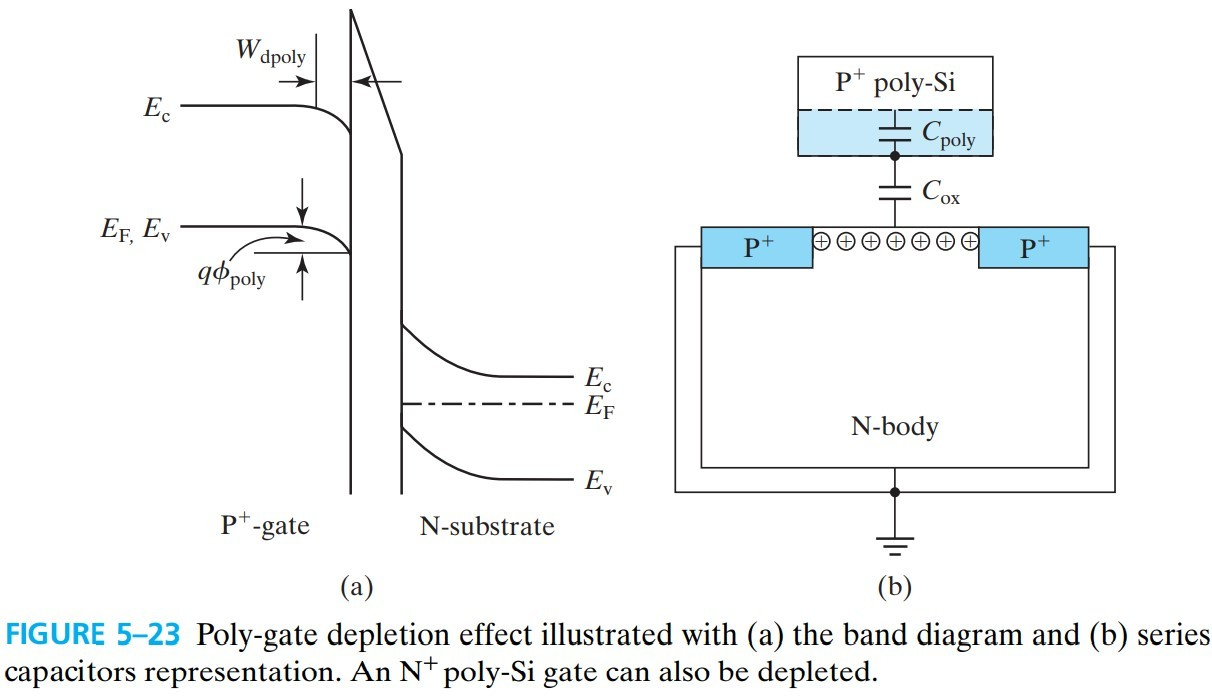gate 的能带存在弯曲，如图 fig 5-23，产生的 poly-Si gate depletion 的厚度为：

$W_\text{dpoly}=\varepsilon_\text{ox} \mathscr{E}_\text{ox}/qN_\text{poly}$

\begin{aligned} C &= \left( \frac{1}{C_\text{ox}}+\frac{1}{C_\text{poly}} \right)^{-1}\\ &= \left( \frac{T_\text{ox}}{\varepsilon_\text{ox}}+\frac{W_\text{dpoly}}{\varepsilon_s} \right)^{-1}\\ &= \frac{\varepsilon_\text{ox}}{T_\text{ox}+W_\text{dpoly}\varepsilon_\text{ox}/\varepsilon_s}\\ &= \frac{\varepsilon_\text{ox}}{T_\text{ox}+W_\text{dpoly}/3} \end{aligned} \tag{5.8.2}

$Q_\text{inv} = -C_\text{ox} (V_g-\phi_\text{poly}-V_t)\\ W_\text{dpoly}=\sqrt{\frac{2\varepsilon_s \phi_\text{poly}}{q N_\text{poly}}}$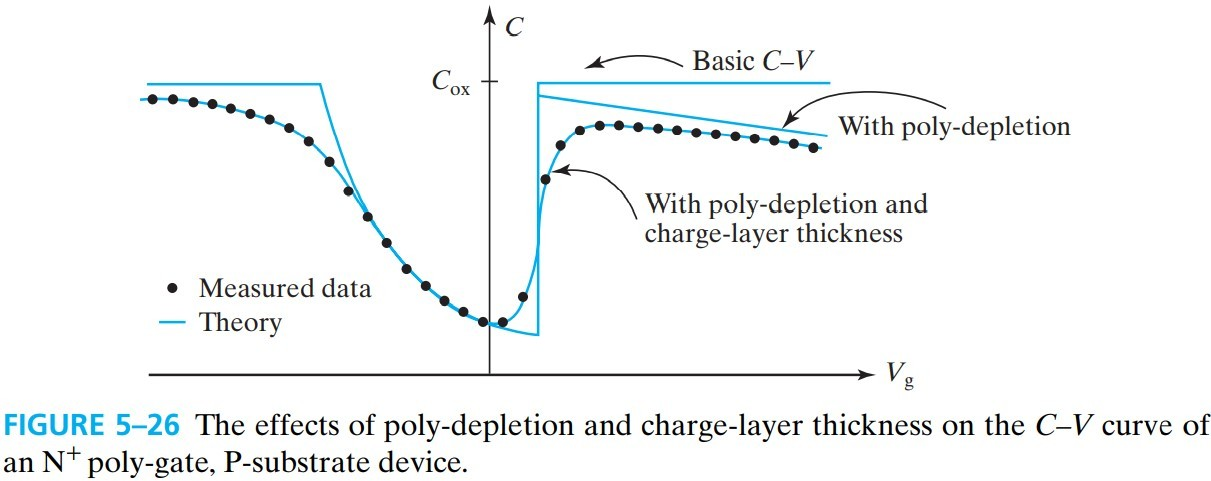• dope the poly-Si heavily
• 坏处：导致杂质渗入 oxide
• substitute the poly-gate with a metal gate（MOSFET）

# 5.9 Charge-Layer Thicknesses and Quantum Mechanical Effect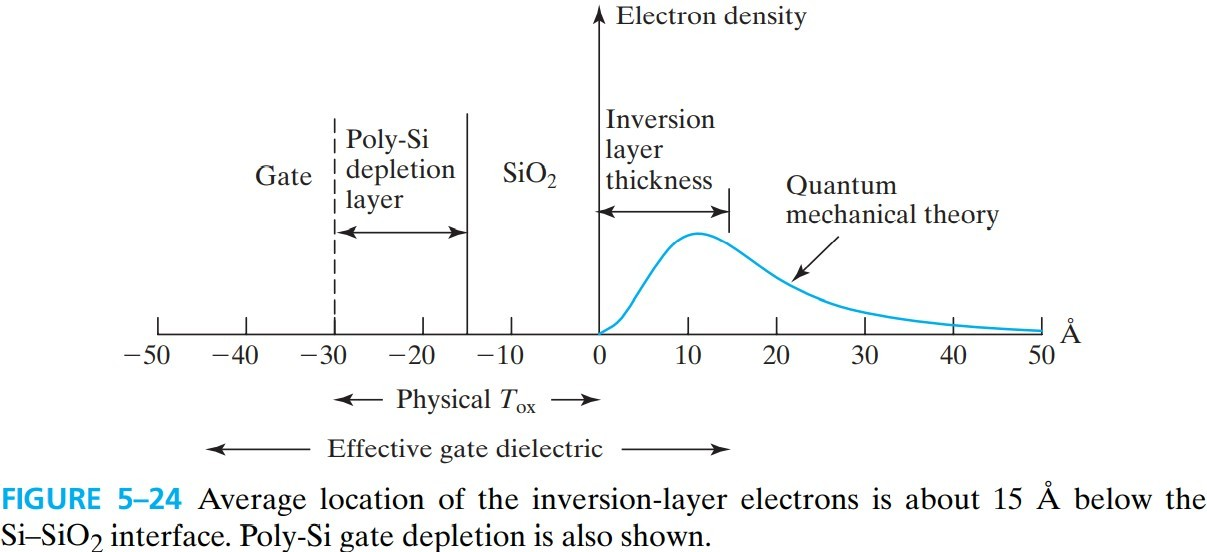$T_\text{oxe}=T_\text{ox}+W_\text{dpoly}/3+T_\text{inv}/3 \tag{5.9.2}$

$T_\text{oxe}$ 称为 electrical oxide thickness，可认为是 total effective oxide thickness，对应的电容称为 effective gate capacitance $C_\text{oxe}$，关系式如下：

\begin{aligned} Q_\text{inv}&=-C_\text{oxe}(V_g-V_t)\\ &=\frac{\varepsilon_\text{ox}}{T_\text{oxe}}(V_g-V_t) \end{aligned} \tag{5.9.3}